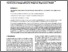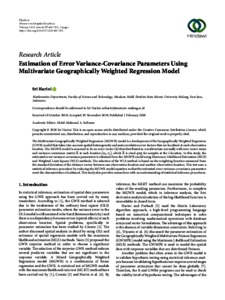# Estimation of error variance-covariance parameters using multivariate geographically weighted regression model

Harini, Sri (2020) Estimation of error variance-covariance parameters using multivariate geographically weighted regression model. Abstract and Applied Analysis, 2020. pp. 1-5. ISSN 1085-3375(print), 1687-0409(online)Preview
Text
Sri Harini 4657151.pdf - Published Version

Full text available at: https://doi.org/10.1155/2020/4657151

## Abstract

The Multivariate Geographically Weighted Regression (MGWR) model is a development of the Geographically Weighted Regression (GWR) model that takes into account spatial heterogeneity and autocorrelation error factors that are localized at each observation location. The MGWR model is assumed to be an error vector that distributed as a multivariate normally with zero vector mean and variance-covariance matrix Σ at each location (ui,vi), which Σ is sized qxq for samples at the i-location. In this study, the estimated error variance-covariance parameters is obtained from the MGWR model using Maximum Likelihood Estimation (MLE) and Weighted Least Square (WLS) methods. The selection of the WLS method is based on the weighting function measured from the standard deviation of the distance vector between one observation location and another observation location. This test uses a statistical inference procedure by reducing the MGWR model equation so that the estimated error variance-covariance parameters meet the characteristics of unbiased. This study also provides researchers with an understanding of statistical inference procedures.

Item Type: Journal Article Heterogeneity; autocorrelation; variance-covariance; weighting function; unbiased 01 MATHEMATICAL SCIENCES > 0104 Statistics > 010405 Statistical Theory Faculty of Mathematics and Sciences > Department of Mathematics Dr Sri Harini 13 Mar 2020 09:19View Item